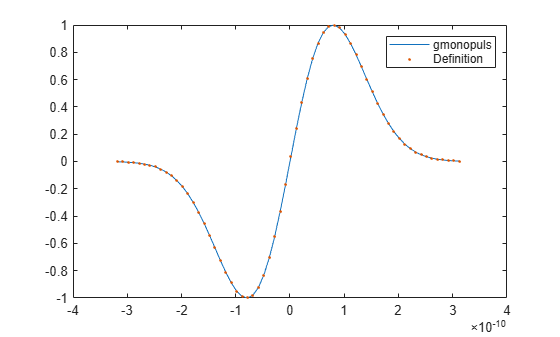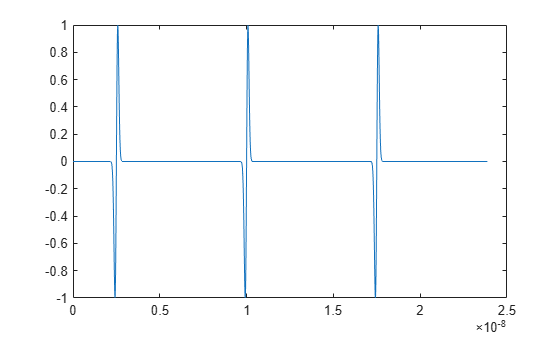# gmonopuls

Gaussian monopulse

## Syntax

``y = gmonopuls(t,fc)``
``tc = gmonopuls('cutoff',fc)``

## Description

example

````y = gmonopuls(t,fc)` returns samples of the unit-amplitude Gaussian monopulse with center frequency `fc` at the times indicated in array `t`.```

example

````tc = gmonopuls('cutoff',fc)` returns the time duration between the maximum and minimum amplitudes of the pulse.```

## Examples

collapse all

Consider a Gaussian monopulse with center frequency ${f}_{c}=2$ GHz and sampled at a rate of 100 GHz. Determine the cutoff time ${t}_{c}$ using the `'cutoff'` option and compute the monopulse between $-2{t}_{c}$ and $2{t}_{c}$.

```fc = 2e9; fs = 100e9; tc = gmonopuls('cutoff',fc); t = -2*tc:1/fs:2*tc; y = gmonopuls(t,fc);```

The monopulse is defined by the equation

`$y\left(t\right)={e}^{1/2}\left(t/\sigma \right)\mathrm{exp}\left(-\left(t/\sigma {\right)}^{2}/2\right),$`

where $\sigma ={t}_{c}/2=1/\left(2\pi {f}_{c}\right)$ and the exponential factor is such that $y\left(\sigma \right)=1$. Plot the two curves and verify that they match.

```sg = 1/(2*pi*fc); ys = exp(1/2)*t/sg.*exp(-(t/sg).^2/2); plot(t,y,t,ys,'.') legend('gmonopuls','Definition')```Consider a Gaussian monopulse with center frequency ${f}_{c}=2$ GHz and sampled at a rate of 100 GHz. Use the monopulse to construct a pulse train with a spacing of 7.5 ns.

Determine the width ${t}_{c}$ of each pulse using the `'cutoff'` option. Set the delay times to be integer multiples of the spacing.

```fc = 2e9; fs = 100e9; tc = gmonopuls('cutoff',fc); D = ((0:2)*7.5+2.5)*1e-9;```

Generate the pulse train such that the total duration is $150{t}_{c}$. Plot the result.

```t = 0:1/fs:150*tc; yp = pulstran(t,D,'gmonopuls',fc); plot(t,yp)```## Input Arguments

collapse all

Time values at which the unit-amplitude Gaussian monopulse is calculated, specified as a vector.

Center frequency, specified as a real positive scalar expressed in hertz. By default, `fc` = `1000` Hz.

## Output Arguments

collapse all

Monopulse of unit amplitude, returned as a vector.

Time duration between the maximum and minimum amplitudes of the pulse, returned as a scalar.

## Tips

Default values are substituted for empty or omitted trailing input arguments.